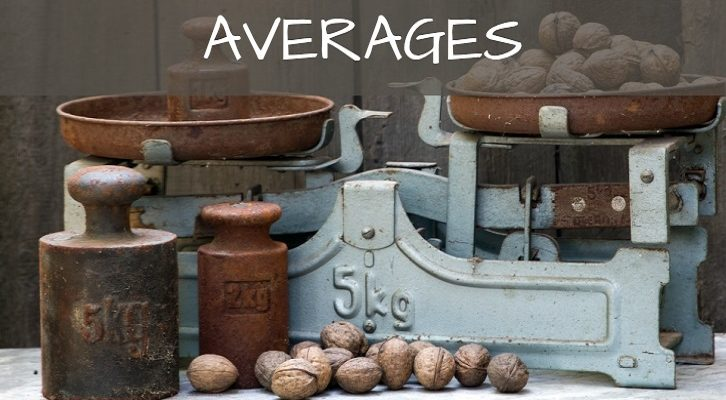# Mean Median – Final few questions

Few more questions on Mean, median. Have just tried to construct a few Yes/No questions to try to build some intuition. These questions are better-answered by constructing examples/counter-examples.

1. The median of n distinct numbers is greater than the average, does this mean that there are more terms above the average than below it?

2. In a sequence of 25 terms, can 20 terms be below the average? Can 20 terms be between median and average?

3. From 10 numbers, a,b,c,…j, all sets of 4 numbers are chosen and their averages computed. Will the average of these averages be equal to the average of the average of the 10 numbers?

There is one further question that does not come under this classification, but an interesting one nevertheless

Q. The 64 squares of a chessboard are filled with natural numbers from 1 to 100. Multiples cells can have the same number (Any number of cells can have the same number). If the value in any square is equal to the average of the numbers in all the squares around it (either 3, 5 or 8 squares, depending on the position of the square), in how many ways can the chessboard be filled?

This is a fairly difficult question, and not exactly a question for CAT prep, but a fun question nevertheless. (This question is an adaptation from one of the Olympiad questions. Have not been able to locate the exact one yet.Will send in a post once I locate the correct Olympiad)

Happy cracking.